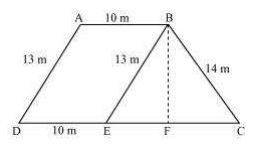# A field is in the shape of a trapezium whose parallel sides are 25 m and 10 m. The non-parallel sides are 14 m and 13 m. Find the area of the field.From the diagram we see that quadrilateral ABED is a parallelogram. So,

AB = ED = 10 m

AD = BE = 13 m

EC = 25-ED = 25-10 = 15 m

From triangle BEC,

Its semi perimeter (s) = (13+14+15)/2 = 21 m

Using Heron’s formula=$$\sqrt{s(s-a)(s-b)(s-c)}$$

Area of ΔBEC =

ar(△ECB) = √s(s-a)(s-b)(s-c)

= √21(21-3)(21-14)(21-15)

= √21×(18)×(7)×(6)

=84 m

We also know that the area of ΔBEC = (½)×CE×BF

84 cm= (½)×15×BF

BF = (168/15) cm = 11.2 cm

So, the total area of ABED will be BF×DE i.e. 11.2×10 = 112 m2

∴ Area of the field = 84+112 = 196 m2